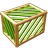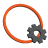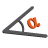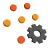Back to Adaptive Vision Library website

You are here: Start » Function Reference » Geometry 3D Features

# Geometry 3D Features

Select a function from the list below.

IconNameDescriptionBox3DCenterReturns the center of the input box in 3D.Box3DCharacteristicPointReturns a characteristic point of a box in 3D.Box3DSurfaceAreaReturns the surface area of the input box in 3D.Box3DVolumeReturns the volume of the input box in 3D.Boxes3DBoundingBox3D_OrNilComputes the bounding box 3D of given boxes in 3D; returns NIL if the array is empty.BoxesBoundingBox3DComputes the bounding box 3D of given boxes in 3D.Circle3DAreaComputes the area of a circle in 3D.Circle3DBoundingBoxComputes the smallest box in 3D containing a circle in 3D.Circle3DPerimeterLengthComputes the length of a circle in 3D perimeter.Plane3DNormalVectorComputes the normal vector of a linePlane3DOrientationComputes the orientation of a plane as angles in the range from 0 to 180 between the plane and coordinate planes.PointsBoundingBox3DComputes the smallest box in 3D containing an array of points in 3D.PointsBoundingBox3D_OrNilComputes the smallest box in 3D containing an array of points in 3D; returns NIL if the array is empty.PointsMassCenter3DComputes the central point of the input points.Segment3DBisectorComputes a plane passing through the center of a segment in 3D at a right angle.Segment3DBoundingBoxComputes the smallest box in 3D containing a segment in 3D.Segment3DCenterComputes the center point of a segment in 3D.Segment3DLengthComputes the length of a segment in 3D.Segment3DLineComputes the line in 3D passing through a segment.Segment3DVectorReturns the vector [x2 - x1, y2 - y1, z2 - z1]Vector3DAzimuthComputes the angle between a 3D vector's projection to the XY plane and the X axis measured toward Y axis, as an angle in the range from 0 to 360.Vector3DElevationComputes the angle between a 3D vector's projection to the XY plane and itself measured toward Z axis, as an angle in the range from -90 to 90.Vector3DLengthComputes the length of a 3D vector.